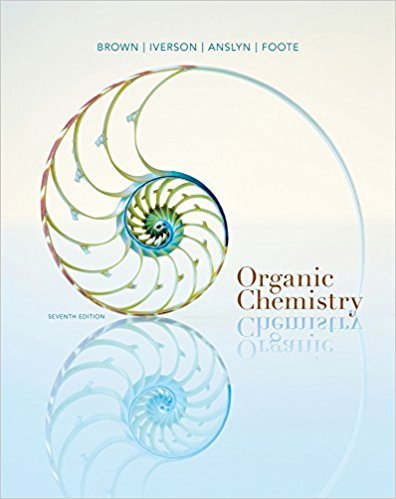×

×

Textbooks / Chemistry / Organic Chemistry 7

# Organic Chemistry 7th Edition - Solutions by Chapter## Full solutions for Organic Chemistry | 7th Edition

ISBN: 9781133952848Organic Chemistry | 7th Edition - Solutions by Chapter

Solutions by Chapter
4 5 0 408 Reviews
##### ISBN: 9781133952848

The full step-by-step solution to problem in Organic Chemistry were answered by , our top Chemistry solution expert on 03/16/18, 05:02PM. This expansive textbook survival guide covers the following chapters: 24. Organic Chemistry was written by and is associated to the ISBN: 9781133952848. This textbook survival guide was created for the textbook: Organic Chemistry, edition: 7. Since problems from 24 chapters in Organic Chemistry have been answered, more than 54478 students have viewed full step-by-step answer.

Key Chemistry Terms and definitions covered in this textbook
• activity series.

A summary of the results of many possible displacement reactions. (4.4)

• amalgam.

An alloy of mercury with another metal or metals. (21.2)

• azo dyes

A class of colored compounds that are formed via azo coupling.

• Bicycloalkane

An alkane containing two rings that share two carbons

• binary compounds.

Compounds formed from just two elements. (2.7)

• carbides.

Ionic compounds containing the C2 22 or C42 ion. (22.3)

• cohesion.

The intermolecular attraction between like molecules. (11.3)

• covalent bond

A bond that results when two atoms share a pair of electrons.

• cycloalkanes

Saturated hydrocarbons of general formula CnH2n in which the carbon atoms form a closed ring. (Section 24.2)

• Dihedral angle

The angle created by two intersecting planes.

• double bond

A covalent bond involving two electron pairs. (Section 8.3)

• ether

A compound with the structure R!O!R.

• fats

Triglycerides that are solids atroom temperature.

• molar mass

The mass of one mole of a substance in grams; it is numerically equal to the formula weight in atomic mass units. (Section 3.4)

• overlap

The extent to which atomic orbitals on different atoms share the same region of space. When the overlap between two orbitals is large, a strong bond may be formed. (Section 9.4)

• percent yield

The ratio of the actual (experimental) yield of a product to its theoretical (calculated) yield, multiplied by 100. (Section 3.7)

• polarimeter

A device that measures the rotation of plane-polarized light caused by optically active compounds.

• protein

A biopolymer formed from amino acids. (Section 24.7)

• resonance

A method that chemists use to deal with the inadequacy of bond-line drawings.